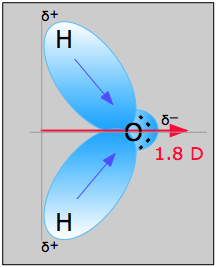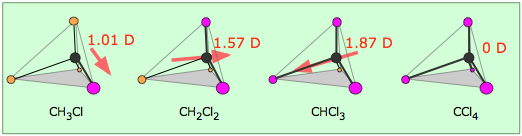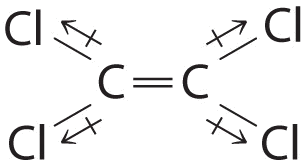# 1.12: Polarity of Molecules

$$\newcommand{\vecs}{\overset { \rightharpoonup} {\mathbf{#1}} }$$ $$\newcommand{\vecd}{\overset{-\!-\!\rightharpoonup}{\vphantom{a}\smash {#1}}}$$$$\newcommand{\id}{\mathrm{id}}$$ $$\newcommand{\Span}{\mathrm{span}}$$ $$\newcommand{\kernel}{\mathrm{null}\,}$$ $$\newcommand{\range}{\mathrm{range}\,}$$ $$\newcommand{\RealPart}{\mathrm{Re}}$$ $$\newcommand{\ImaginaryPart}{\mathrm{Im}}$$ $$\newcommand{\Argument}{\mathrm{Arg}}$$ $$\newcommand{\norm}{\| #1 \|}$$ $$\newcommand{\inner}{\langle #1, #2 \rangle}$$ $$\newcommand{\Span}{\mathrm{span}}$$ $$\newcommand{\id}{\mathrm{id}}$$ $$\newcommand{\Span}{\mathrm{span}}$$ $$\newcommand{\kernel}{\mathrm{null}\,}$$ $$\newcommand{\range}{\mathrm{range}\,}$$ $$\newcommand{\RealPart}{\mathrm{Re}}$$ $$\newcommand{\ImaginaryPart}{\mathrm{Im}}$$ $$\newcommand{\Argument}{\mathrm{Arg}}$$ $$\newcommand{\norm}{\| #1 \|}$$ $$\newcommand{\inner}{\langle #1, #2 \rangle}$$ $$\newcommand{\Span}{\mathrm{span}}$$$$\newcommand{\AA}{\unicode[.8,0]{x212B}}$$

Learning Objectives

After completing this section, you should be able to

1. explain how dipole moments depend on both molecular shape and bond polarity.
2. predict whether a molecule will possess a dipole moment from the molecular formula or structure.
3. use the presence or absence of a dipole moment as an aid to deducing the structure of a given compound.

Key Terms

Make certain that you can define, and use in context, the key term below.

• dipole moment

Study Notes

You must be able to combine your knowledge of molecular shapes and bond polarities to determine whether or not a given compound will have a dipole moment. Conversely, the presence or absence of a dipole moment may also give an important clue to a compound’s structure. BCl3, for example, has no dipole moment, while NH3 does. This suggests that in BCl3 the chlorines around boron are in a trigonal planar arrangement, while the hydrogens around nitrogen in NH3 have a less symmetrical arrangement - trigonal pyramidal.

Remember that the C-H bond is assumed to be non-polar.

### Molecular Dipole Moments

In molecules containing more than one polar bond, the molecular dipole moment is just the vector combination of what can be regarded as individual "bond dipole moments".  Mathematically, dipole moments are vectors; they possess both a magnitude and a direction. The dipole moment of a molecule is therefore the vector sum of the dipole moments of the individual bonds in the molecule. If the individual bond dipole moments cancel one another, there is no net dipole moment. Such is the case for CO2, a linear molecule (Figure $$\PageIndex{1a}$$). Each C–O bond in CO2 is polar, yet experiments show that the CO2 molecule has no dipole moment. Because the two C–O bond dipoles in CO2 are equal in magnitude and oriented at 180° to each other, they cancel. As a result, the CO2 molecule has no net dipole moment even though it has a substantial separation of charge. In contrast, the H2O molecule is not linear (Figure $$\PageIndex{1b}$$); it is bent in three-dimensional space, so the dipole moments do not cancel each other. Thus a molecule such as H2O has a net dipole moment. We expect the concentration of negative charge to be on the oxygen, the more electronegative atom, and positive charge on the two hydrogens. This charge polarization allows H2O to hydrogen-bond to other polarized or charged species, including other water molecules.Figure $$\PageIndex{1}$$: How Individual Bond Dipole Moments Are Added Together to Give an Overall Molecular Dipole Moment for Two Triatomic Molecules with Different Structures. (a) In CO2, the C–O bond dipoles are equal in magnitude but oriented in opposite directions (at 180°). Their vector sum is zero, so CO2 therefore has no net dipole. (b) In H2O, the O–H bond dipoles are also equal in magnitude, but they are oriented at 104.5° to each other. Hence the vector sum is not zero, and H2O has a net dipole moment.

The following is a simplified equation for a simple separated two-charge system that is present in diatomic molecules or when considering a bond dipole within a molecule.

$\mu_{diatomic} = Q \times r \label{1a}$

This bond dipole, µ (Greek mu) is interpreted as the dipole from a charge separation over a distance $$r$$ between the partial charges $$Q^+$$ and $$Q^-$$ (or the more commonly used terms $$δ^+$$ - $$δ^-$$); the orientation of the dipole is along the axis of the bond. The units on dipole moments are typically debyes (D) where one debye is equal to 3.336 x 1030 coulomb meters (C · m) in SI units. Consider a simple system of a single electron and proton separated by a fix distance. The unit charge on an electron is 1.60 X 1019 C and the proton & electron are 100 pm apart (about the length of a typical covalent bond), the dipole moment is calculated as:

\begin{align} \mu &= Qr \nonumber \\[4pt] &= (1.60 \times 10^{-19}\, C)(1.00 \times 10^{-10} \,m) \nonumber \\[4pt] &= 1.60 \times 10^{-29} \,C \cdot m \label{2} \end{align}

\begin{align} \mu &= (1.60 \times 10^{-29}\, C \cdot m) \left(\dfrac{1 \;D}{3.336 \times 10^{-30} \, C \cdot m} \right) \nonumber \\[4pt] &= 4.80\; D \label{3} \end{align}

$$4.80\; D$$ is a key reference value and represents a pure charge of +1 and -1 separated by 100 pm. However, if the charge separation were increased then the dipole moment increases (linearly):

• If the proton and electron were separated by 120 pm:

$\mu = \dfrac{120}{100}(4.80\;D) = 5.76\, D \label{4a}$

• If the proton and electron were separated by 150 pm:

$\mu = \dfrac{150}{100}(4.80 \; D) = 7.20\, D \label{4b}$

• If the proton and electron were separated by 200 pm:

$\mu = \dfrac{200}{100}(4.80 \; D) = 9.60 \,D \label{4c}$

Example $$\PageIndex{1}$$: Water

The water molecule in Figure $$\PageIndex{1}$$ can be used to determine the direction and magnitude of the dipole moment. From the electronegativities of oxygen and hydrogen, the difference is 1.2e for each of the hydrogen-oxygen bonds. Next, because the oxygen is the more electronegative atom, it exerts a greater pull on the shared electrons; it also has two lone pairs of electrons. From this, it can be concluded that the dipole moment points from between the two hydrogen atoms toward the oxygen atom. Using the equation above, the dipole moment is calculated to be 1.85 D by multiplying the distance between the oxygen and hydrogen atoms by the charge difference between them and then finding the components of each that point in the direction of the net dipole moment (the angle of the molecule is 104.5˚).

The bond moment of O-H bond =1.5 D, so the net dipole moment is

$\mu=2(1.5) \cos \left(\dfrac{104.5˚}{2}\right)=1.84\; D \nonumber$### Dipoles with Differing Atoms

In more complex molecules with more than one polar covalent bonds, the three-dimensional geometry and the compound’s symmetry determine whether the molecule has a net dipole moment. The manner in which the individual bonds contribute to the dipole moment of the molecule is nicely illustrated by the series of chloromethanes shown below.  The electronegative chlorine draws electrons towards itself.Consider $$CCl_4$$, (left panel in figure below), which as a molecule is not polar - in the sense that it doesn't have an end (or a side) which is slightly negative and one which is slightly positive. The whole of the outside of the molecule is somewhat negative, but there is no overall separation of charge from top to bottom, or from left to right. In contrast, $$CHCl_3$$ is a polar molecule (right panel in figure above). However, although a molecule like CHCl3 has a tetrahedral geometry, the atoms bonded to carbon are not identical. Consequently, the bond dipole moments do not cancel one another, and the result is a molecule which has a dipole moment.  The hydrogen at the top of the molecule is less electronegative than carbon and so is slightly positive. This means that the molecule now has a slightly positive "top" and a slightly negative "bottom", and so is overall a polar molecule.Figure $$\PageIndex{3}$$: Bond polarities for CCl(left) and CHCl3(right)

Electron densities in a molecule (and the dipole moments that unbalanced electron distributions can produce) are easily visualized with electrostatic potential maps. In this example, $$CHCl_3$$, the blue area centered on carbon represents a electron-deficient positive area, of the molecule, the red area centered on the chlorine, represents a electron-abundant negative area. This charge separation creates a net dipole moment of 1.87 D which points in the direction of the chlorine. In, $$CCl_4$$ the evenly spaced red areas represent that there is no separation of charge in the molecule. $$CCl_4$$ has a net dipole moment of zero which makes it a nonpolar molecule.chloromethane tetrachloromethane (μ = 1.87 D) (μ = 0 D)

Figure $$\PageIndex{4}$$: Electrostatic potential maps and dipole moments for chloromethane and tetrachloromethane (carbon tetrachloride).

Molecules with asymmetrical charge distributions have a net dipole momentOther examples of molecules with polar bonds are shown in Figure $$\PageIndex{2}$$. In molecules like BCl3 and CCl4, that have only one type of bond and a molecular geometries that are highly symmetrical (trigonal planar and tetrahedral), the individual bond dipole moments completely cancel, and there is no net dipole moment. However, although a molecule like CHCl3 has a tetrahedral geometry, the atoms bonded to carbon are not identical. Consequently, the bond dipole moments do not cancel one another, and the result is a molecule which has a dipole moment.net dipole no net dipole net dipole net dipole no net dipole net dipole no net dipole no net dipole HCl BCl3 CH2O NH3 CCl4 CHCl3 PF5 SF6

Figure $$\PageIndex{5}$$: Molecules with Polar Bonds. Individual bond dipole moments are indicated in black. Due to their different three-dimensional structures, some molecules with polar bonds have a net dipole moment (HCl, CH2O, NH3, and CHCl3), indicated in red, whereas others do not because the bond dipole moments cancel (BCl3, CCl4, PF5, and SF6).

Table $$\PageIndex{1}$$: Dipole Moments of Some Compounds
Compound Dipole Moment (Debyes)
NaCl 9.0 (measured in the gas phase)
CH2O 2.33
CH3Cl 1.87
H2O 1.85
CH3OH 1.70
NH3 1.47
CH3NH2 1.31
CO2 0
CCl4 0
CH4 0
CH3CH3 0

The table above give the dipole moment of some common substances.  Sodium chloride has the largest dipole listed (9.00 D) because it is an ionic compounds. Even small organic compounds such as formaldehyde (CH2O, 2.33 D) and methanol (CH3OH, 1.70 D) have significant dipole moments.  Both of these molecules contain the strongly electronegative oxygen atom lone pair electrons which gives rise to considerable dipole moments.methanol formaldehyde (μ = 1.70 D) (μ = 2.33 D)

In contrast many organic molecule have a zero dipole moment despite the fact that they are made up of polar covalent bonds.  In, structures with highly symmetrical molecular geometries, the polar bonds and the lone pair electrons can can exactly cancel leaving no overall charge separation.carbon dioxide carbon tetrachloride ethane (μ = 0 D) (μ = 0 D) (μ = 0 D)

Exercise $$\PageIndex{1}$$

Which of the molecules below have molecular dipole moments?Only molecule (b) does not have a molecular dipole, due to its symmetry (bond dipoles are equal and in opposite directions). Add texts here.

Exercise $$\PageIndex{2}$$

Draw out the line structure of the molecule with a molecular formula of C2Cl4. Indicate all of the individual bond polarities and predict if the molecule is polar or nonpolar.

Although the C–Cl bonds are rather polar, the individual bond dipoles cancel one another in this symmetrical structure, andExercise $$\PageIndex{3}$$

The following molecule has no net dipole moment, explain.The hydroxyl groups are oriented opposite of one another and therefore the dipole moments would “cancel” one another out. Therefore having a zero net-dipole.

Exercise $$\PageIndex{4}$$

Within reactions with carbonyls, such as a hydride reduction reaction, the carbonyl is attacked from the carbon side and not the oxygen side. Using knowledge of electronegativity explain why this happens.The oxygen is more electronegative than the carbon and therefore creates a dipole along the bond. This leads to having a partial positive charge on the carbon and the reduction can take place.Exercise $$\PageIndex{1}$$

Which molecule(s) has a net dipole moment?

1. H2S
2. NHF2
3. BF3

Strategy:

For each three-dimensional molecular geometry, predict whether the bond dipoles cancel. If they do not, then the molecule has a net dipole moment.

Solution:

The total number of electrons around the central atom, S, is eight, which gives four electron pairs. Two of these electron pairs are bonding pairs and two are lone pairs, so the molecular geometry of H2S is bent. The bond dipoles cannot cancel one another, so the molecule has a net dipole moment.Difluoroamine has a trigonal pyramidal molecular geometry. Because there is one hydrogen and two fluorines, and because of the lone pair of electrons on nitrogen, the molecule is not symmetrical, and the bond dipoles of NHF2 cannot cancel one another. This means that NHF2 has a net dipole moment. We expect polarization from the two fluorine atoms, the most electronegative atoms in the periodic table, to have a greater affect on the net dipole moment than polarization from the lone pair of electrons on nitrogen.• The molecular geometry of BF3 is trigonal planar. Because all the B–F bonds are equal and the molecule is highly symmetrical, the dipoles cancel one another in three-dimensional space. Thus BF3 has a net dipole moment of zero:

## Contributors

This page titled 1.12: Polarity of Molecules is shared under a not declared license and was authored, remixed, and/or curated by Layne Morsch.